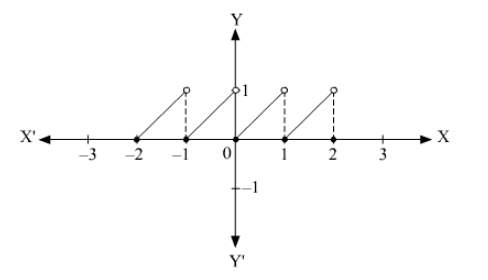# The set of points where f(x)

Question:

The set of points where f(x) = x – [x] is discontinuous is _____________.

Solution:

The graph of f(x) = x – [x] is shown below.It can be seen that, the function f(x) = x – [x] is discontinuous at all integral values of x. So, the set of point where where f(x) = x – [x] is discontinuous is i.e. the set of integers.

The set of points where f(x) = x – [x] is discontinuous is _____ the set of integers i.e.  Z______.